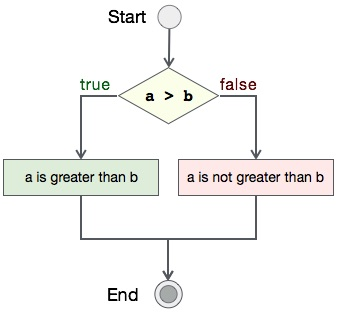# Program to append file in C

## Algorithm

Let's first see what should be the step-by-step procedure to compare two integers−

```START

Step 1 → Take two integer variables, say A & B

Step 2 → Assign values to variables

Step 3 → Compare variables if A is greater than B

Step 4 → If true print A is greater than B

Step 5 → If false print A is not greater than B

STOP
```

## Flow Diagram

We can draw a flow diagram for this program as given below −## Pseudocode

Let's now see the pseudocode of this algorithm −

```procedure compare(A, B)

IF A is greater than B
DISPLAY "A is greater than B"
ELSE
DISPLAY "A is not greater than B"
END IF

end procedure
```

## Implementation

Assuming we have file with name file_append.txt with this content −

```This text was already there in the file.
```

Now, we shall see the actual implementation of the program −

```#include <stdio.h>

int main()
{
FILE *fp;
char ch;
char *filename = "file_append.txt";
char *content = "This text is appeneded later to the file, using C programming.";

/*  open for writing */
fp = fopen(filename, "r");

printf("\nContents of %s  -\n\n", filename);

while ((ch = fgetc(fp) )!= EOF)
{
printf ("%c", ch);
}

fclose(fp);

fp = fopen(filename, "a");

/* Write content to file */
fprintf(fp, "%s\n", content);

fclose(fp);

fp = fopen(filename, "r");

printf("\nContents of %s  -\n", filename);

while ((ch = fgetc(fp) )!= EOF)
{
printf ("%c", ch);
}

fclose(fp);

return 0;
}
```

## Output

Output of this program should be −

```Contents of file_append.txt  -
This text was already there in the file.

Appending content to file_append.txt...

Content of file_append.txt after 'append' operation is -

This text was already there in the file.
This text is appeneded later to the file, using C programming.
```